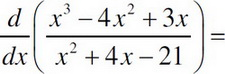# AP Calculus AB Practice Test 21

### Test Information10 questions20 minutes

Calculator Disallowed

1. Find the second derivative of x2y = 2.

2. If y = ln(6x3 - 2x2), then f′(x)=

3. Find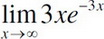.

4. The radius of a sphere is measured to be 5 cm with an error of ± 0.1 cm. Use differentials to approximate the error in the volume.

5. A side of a cube is measured to be 10 cm. Estimate the change in surface area of the cube when the side shrinks to 9.8 cm.

6. Find the derivative of y, when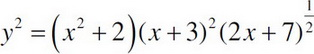at (1, 12) ?

7.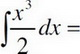8.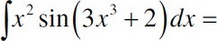9. If f(x) =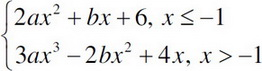and is differentiable for all real values, then b = ?

10.